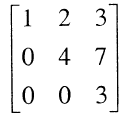# GATE | GATE CS 2011 | Question 40

Consider the matrix as given below.Which one of the following options provides the CORRECT values of the eigenvalues of the matrix?
(A) 1, 4, 3
(B) 3, 7, 3
(C) 7, 3, 2
(D) 1, 2, 3

Explanation:

The Eigen values of a triangular matrix are given by its diagonal entries. We can also calculate (or verify given answers) using characteristic equation obtained by |M – λI| = 0.

1-λ    2     3

0     4-λ    7           =       0

0      0     3-λ

Which means

(1-λ)(4-λ)(3-λ) = 0

My Personal Notes arrow_drop_up
Article Tags :

Be the First to upvote.

Please write to us at contribute@geeksforgeeks.org to report any issue with the above content.